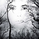6776 views
MARKET DELTA INDICATOR v0.5 beta

Market Delta is suitable for daytrading on intraday timeframes, is a volume based indicator which allows to see the UP VOLUME vs the DOWN VOLUME , the DELTA (difference) and the CUMULATIVE DELTA (cumulative sum of difference) between them
This indicator is based on contracts volume (data avaiable), not in ask/bid volume (data not avaiable)

The up/down volume is calculated at each candle as follows:
- calculate the ticks of the range, top wick and bottom wick
- calculate the ticks up and ticks down to get the total ticks of the candle
- calculate the volume per tick as total volume divided by total ticks
- calculate the up and down volume as volume per tick multiplied by up ticks and down ticks
The delta is calculated as volume up minus volume down
The cumulative delta is a cumulative sum of delta and is resetted to 0 twice a day at the globex open and at the us cash open

By default the indicator plots the 'CANDLE MODE' which is useful for charting the cumulative volume to find out support and resistance zones where the volume is rejected or pass thru, as the volume moves so does the price, price always follows the volume , price goes away from where volume dries and price auctions comfortable where is plenty of volume , in a way PRICE FEEDS ON VOLUME
An indication about the plotting style in the volume , delta and cumulative delta modes: I can't use histogram as intended due a bug at autoresizing the scale in the candle mode, so the styles used are areabr and circles.

FEATURES
- Plot volume in one of four modes: Volume Up/Down, Delta, Cumulative Delta, Cumulative Delta as Candles
- Cumulative delta resetted twice a day (globex and cash open)
- Show a base line at 0

SETTINGS
- Mode: select one of the four volume output modes: Volume , Delta, Cumulative Delta and Candles. Candles by default
- Show zero line: show/hide the zero base line. False by default.

HOW TO SETTING UP THE INDICATOR:
BE AWARE, by default the indicator settings are configured for using the Cumulative Delta Candle Mode
- Candles Mode Settings: configured by default, mode candles and zero line off
- Volume , Delta, Cumulative Delta Mode Settings: select the mode you want and switch on/off the zero line

Open-source script

In true TradingView spirit, the author of this script has published it open-source, so traders can understand and verify it. Cheers to the author! You may use it for free, but reuse of this code in a publication is governed by House Rules. You can favorite it to use it on a chart.

Want to use this script on a chart?changed to take into account gaps and be able to watch daily charts
(It turns out that you cannot insert square brackets here, so I inserted curly braces instead of square)

//@version=4
study("Volume_Delta",shorttitle='VD')

// volume & delta calculus
gapUp= open>close{1}?(open-close{1})/syminfo.mintick:0
gapDown= open<close{1}?(close{1}-open)/syminfo.mintick:0
candleRange = (high-low)/syminfo.mintick
candleTopWick = open>close?(high-open)/syminfo.mintick:(high-close)/syminfo.mintick
candleBottomWick = open>close?(close-low)/syminfo.mintick:(open-low)/syminfo.mintick
ticksUp = open>close?candleTopWick+candleBottomWick+gapUp:candleRange+gapUp
ticksDown = open>close?candleRange+gapDown:candleTopWick+candleBottomWick+gapDown
totalTicks = ticksUp+ticksDown
volByTick = totalTicks==0?0:volume/totalTicks
volUp = ticksUp*volByTick
volDown = ticksDown*volByTick
delta = volUp-volDown

cumDelta = 0.0
cumDelta := nz(cumDelta{1})+delta

// getting ohlc values to plot the cumulative delta candles.
o = cumDelta{1}
c = cumDelta
h = delta>=0 ? c : o
l = delta<=0 ? c : o

// plotting cumulative delta as candles. Two 'plotcandle' calls to set wickcolor/bordercolor 'dinamically' setting 'o' to 'na' when needed
plotcandle(o<=c?o:na, h, l, c, title='Cumulative Delta Green Candle', color = color.green,wickcolor=color.green,bordercolor=color.green)
plotcandle(o>=c?o:na, h, l, c, title='Cumulative Delta Red Candle', color = color.red,wickcolor=color.red,bordercolor=color.red)